[Introduction]  [Signal arithmetic]  [Signals and noise]   [Smoothing]   [Differentiation]  [Peak Sharpening]  [Harmonic analysis]   [Fourier convolution]  [Fourier deconvolution]  [Fourier filter]  [Wavelets]   [Peak area measurement]  [Linear Least Squares]  [Multicomponent Spectroscopy]  [Iterative Curve Fitting]  [Hyperlinear quantitative absorption spectrophotometry] [Appendix and Case Studies]  [Peak Finding and Measurement]  [iPeak]   [iSignal]  [Peak Fitters]   [iFilter]  [iPower]  [List of downloadable software]  [Interactive tools]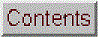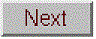## Curve fitting C: Non-linear Iterative Curve Fitting (a.k.a. "spectral deconvolution" or "peak deconvolution")

[Basics]  [Spreadsheets]  [Matlab and Octave]  [Fitting peaks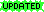]  [Peak fitter functions]  [Accuracy and precision of peak parameter measurement]  [Exponential broadening]   [Effect of smoothing]  [Interactive tools

The linear least squares curve fitting described in "Curve Fitting A" is simple and fast, but it is limited to situations where the dependent variable can be modeled as a polynomial with linear coefficients. We saw that in some cases a non-linear situation can be converted into a linear one by a coordinate transformation, but this is possible only in some special cases, it may restrict the range of allowable dependent data values, and, in any case, the resulting coordinate transformation of the noise in the data can result in imprecision in the parameters measured in this way.

The most general way of fitting any model to a set of data is the iterative method, a kind of "trial and error" procedure in which the parameters of the model are adjusted in a systematic fashion until the equation fits the data as close as required. This sounds like a brute-force approach, and it is. In fact, in the days before computers, this method was only grudgingly applied. But its great generality, coupled with advances in computer speed and algorithm efficiency, means that iterative methods are more widely used now than ever before.

Iterative methods proceed in the following general way:
(1) Select a model for the data;
(2) Make first "guesses" ("start" values) of all the non-linear parameters (i.e. position and width; there is no need to guess the peak heights).;
(3) A computer program computes the model and compares it to the data set, calculating a fitting error;
(4) If the fitting error is greater than the required fitting accuracy, the program systematically changes the parameters and loops back around to the previous step and repeats until the required fitting accuracy is achieved or the maximum number or iterations is reached.
This continues until the fitting error is less than the specified error. One popular technique for doing this is called the Nelder-Mead Modified Simplex. This is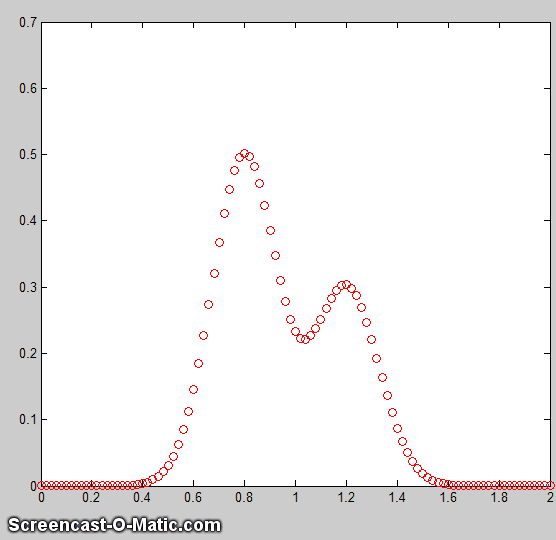essentially a way of organizing and optimizing the changes in parameters (step 4, above) to shorten the time required to fit the function to the required degree of accuracy. With contemporary personal computers, the entire process typically takes only a fraction of a second to a few seconds, depending on the complexity of the model and the number of independently adjustable parameters in the model. The animation on the right (script) shows the working of the iterative process for a 2-peak unconstrained Gaussian fit to a small set of x,y data. This model has four nonlinear variables (the positions and width of the two Gaussians, which are determined by iteration) and two linear variables (the peak heights of the two Gaussians, which are determined directly by regression for each trial iteration). In order to allow the process to be observed in action, this animation is slowed down artificially by (a) plotting step-by-step, (b) making a bad initial guess, and (c) adding a "pause()" statement. Without all that slowing it down, the process normally takes only about 0.05 seconds on a standard desktop PC, depending mainly on the number of nonlinear variables that must be iterated. This even works if the peaks are so overlapped that they bend into a single peak, as shown by Demofitgauss2AnimatedBlended.m, but it may take more iterations and it's more sensitive to any random noise in the data (which you can set in line 13).

Note: the script that created this animation, Demofitgauss2animated.m, requires the gaussian.m, fitgauss2animated.m, and fminsearch.m functions to be in the Matlab/Octave path. A modified version of this script, Demofitgauss2animatedError.m, also plots the fitting error vs iteration number, showing that a poor initial guess (start value) can send the cause the routine to wander all over the place, requiring many more iterations (and more time) to find a good fit, which is not very efficient from the point of view of a human observer like yourself who can visually estimate the peak parameters much more intelligently. A good guess requires fewer iterations. One way to get very good start values is to use a fast, single-pass peak detector algorithm to determine the number of peaks and their approximate positions and widths (as will be covered in a later section), but that will only work when the peaks to be fit have distinct maxima and are not blended into a single peak.
And as you might expect, a 3-peak model, which has six non-linear parameters to optimize, will take even more iterations.

The main difficulty of the iterative methods is that they sometime fail to converge at an overall optimum solution in difficult cases, especially if given a bad initial starting guesses. To understand how that could happen, think of an analogy in physical terms: you deposit a blind man on a hilly landscape and ask him to walk to the highest peak, given only a stick to probe his immediate surroundings to see if the ground is higher or lower on each side. If it's higher, he walks there and probes around that area. He stops when all the areas around him are lower than where he is. This works well if there is one smooth hill. But what if there are multiple small hills, of if the ground is rocky and uneven?  He is very likely to stop at one of the smaller hills, not knowing that there might be an even higher hill nearby.

The standard approach to handle the problem of "local solutions" is to restart the algorithm with another set of first guesses; repeat that several times, remember each result, and take the one with the lowest fitting error (that process is automated in the peak fitting functions described below). Iterative curve fitting also takes longer than linear regression - with typical modern personal computers, an iterative fit might take fractions of a second where a regression would take fractions of a millisecond. Still, this is fast enough for many purposes.

The accuracy and precision of the model parameters measured by iterative fitting, like classical least-squares fitting, depends strongly on a good model, accurate compensation for the background/baseline, the signal-to-noise ratio, and the number of data points across the feature that you wish to measure. It is not practical to predict the standard deviations of the measured model parameters using the algebraic approach, but both the Monte Carlo simulation and bootstrap methods are applicable.

Note: the term "spectral deconvolution" or "band deconvolution" is often used to refer to this technique, but in this essay, "deconvolution"
specifically means Fourier deconvolution, an independent concept that is treated in another section. In Fourier deconvolution, the underlying peak shape is unknown but the broadening function is assumed to be known; whereas in iterative least-squares curve fitting it's just the reverse: the peak shape must be known but the width of the broadening process, which determines the width and shape of the peaks in the recorded data, is unknown. Thus the term "spectral deconvolution" is ambiguous: it might mean the Fourier deconvolution of a response function from a spectrum, or it might mean the decomposing of a spectrum into its separate additive peak components. These are different processes; don't get them confused. See Appendix H: Fourier deconvolution vs curve fitting.

Spreadsheets and stand-alone programs. Both Excel and OpenOffice Calc have a
"Solver" capability that will change specified cells in an attempt to produce a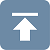specified goal; this can be used in peak fitting to minimize the fitting error between a set of data and a proposed calculated model, such as a set of overlapping Gaussian bands. (This external source, https://www.wallstreetmojo.com/solver-in-excel/, has a detailed graphical explanation of using the Solver.) Solver includes three different solving methods. This Excel spreadsheet example (screen shot) demonstrates how this is used to fit four Gaussian components to a sample set of x,y data that has already been entered into columns A and B, rows 22 to 101 (you could type or paste in your own data there).   After entering the data, do a visual estimate of how many Gaussian peaks it might take to represent the data, and their locations and widths, and type those values into the 'Proposed model' table. The spreadsheet calculates the best-fit values for the peak heights (by multilinear regression) in the 'Calculated amplitudes' table and plots the data and the fit. It also plots the "residuals", which are the point-by-point differences between the data and the model; ideally the residuals would be zero, or at least small. (Adjust the x-axis scale of these graphs to fit your data). The next step is to use Solver function to "fine-tune" the position and width of each component to minimize the % fitting error (in red) and to make the residuals plot as random as possible: click Data in the top menu bar, click Solver (upper right) to open the Solver box, into which you type "C12" into "Set Objective", click "min", select the cells in the "Proposed Model" that you want to optimize, add any desired constraints in the "Subject to the Constraints" box, and click the Solve button. The position, width, and amplitude of all the components are automatically optimized by Solver and best fit is displayed. (You can see that the Solver has changed the selected entries in the proposed model table, reduced the fitting error (cell C12, in red), and made the residuals smaller and more random). If the fit fails, change the starting values, click Solver, and click the Solve button. You can automate the above process and reduce it to a single function-key press by using macros, as described in Appendix N.

So, how many Gaussian components does it take to fit the data? One way to tell is to look at the plot of the residuals (which shows the point-by-
point difference between the data and the fitted model), and add components until the residuals are random, not wavy, but this works only if the data are not smoothed before fitting. Here's an example - a set of real data that are fit with an increasing sequence of two Gaussians, three Gaussians, four Gaussians, and five Gaussians. As you look at this sequence of screenshots, you'll see the percent fitting error decrease, the R2 value become closer to 1.000, and the residuals become smaller and more random. (Note that in the 5-component fit, the first and last components are not peaks within the 250-600 x range of the data, but rather account for the background). There is no need to try a 6-component fit because the residuals are already random at 5 components and more components than that would just "fit the noise" and would likely be unstable and give a very different result with another sample of that signal with different noise.

There are a number of downloadable non-linear iterative curve fitting adds-ons and macros for Excel and OpenOffice. For example, Dr. Roger Nix of Queen Mary University of London has developed a very nice Excel/VBA spreadsheet for curve fitting X-ray photoelectron spectroscopy (XPS) data, but it could be used to fit other types of spectroscopic data. A 4-page instruction sheet is also provided.

There are also
many examples of stand-alone freeware and commercial programs, including PeakFit, Data Master 2003, MyCurveFit, Curve Expert, Origin, ndcurvemaster, and the R language.

If you use a spreadsheet for this type of curve fitting, you have to build a custom spreadsheet for each problem, with the right number of rows for the data and with the desired number of components. For
example, my CurveFitter.xlsx template is only for a 100-point signal and a 5-component Gaussian model. It's easy to extend to a larger number of data points by inserting rows between 22 and 100, columns A through N, and drag-copying the formulas down into the new cells (e.g. CurveFitter2.xlsx is extended to 256 points). To handle other numbers of components or model shapes you would have to insert or delete columns between C and G and between Q and U and edit the formulas, as has been done in this set of templates for , and 6 Gaussians.

If your peaks are superimposed on a baseline, you can include a model for the baseline as one of the components. For instance, if you wish to fit 2 Gaussian peaks on a linear tilted slope baseline, select a 3-component spreadsheet template and change one of the Gaussian components to the equation for a straight line (y=mx+b, where m is the slope and b is the intercept). A template for that particular case is CurveFitter2GaussianBaseline.xlsx (graphic); don't click "Make Unconstrained Variables Non-Negative" in this case, because the baseline model may well need negative variables, as it does in this particular example. If you want to use another peak shape or another baseline shape, you'd have to modify the equation in row 22 of the corresponding columns C through G and drag-copy the modified cell down to the last row, as was done to change  the Gaussian peak shape into a Lorentzian shape in CurveFitter6Lorentzian.xlsx. Or you could make columns C through G contain equations for different peak or baseline shapes. For exponentially broadened Gaussian peak shapes, you can use CurveFitter2ExpGaussianTemplate.xlsx for two overlapping peaks (screen graphic). In this case, each peak has four parameters: height, position, width, and lambda (which determines the asymmetry - the extent of exponential broadening).

In some cases, it is useful to add constraints to the variables determined by iteration, for example to constrain them to be greater than zero, or constrained between two limits, or equal to each other, etc. Doing so will force the solutions to adhere to known expectations and avoid nonphysical solutions. This is especially important for complex shapes such as the
exponentially broadened Gaussian just discussed in the previous paragraph. You can do this by adding those constraints using the "Subject to the Constraints:" box in the center of the "Solver Parameters" box (see the graphic above). For details, see https://www.solver.com/excel-solver-add-change-or-delete-constraint?

Matlab and Octave have a convenient and efficient function called "fminsearch" that uses the Nelder-Mead method. It was originally designed for finding theminimum values of functions, but it can be applied to least-squares curve fitting by creating an anonymous function (a.k.a. "lambda" function) that computes the model, compares it to the data, and returns the fitting error. For example, writing parameters = fminsearch(@(lambda)(fitfunction(lambda,x,y)),start) performs an iterative fit of the data in the vectors x,y to a model described in a previously-created function called fitfunction, using the first guesses in the vector start. The parameters of the best-fit model are returned in the vector "parameters", in the same order that they appear in "start".

Note: for Octave users, the fminsearch function is contained in the "Optim" add-on package (use the latest version 1.2.2), downloadable from Octave Forge.  It is a good idea to install all these add-on packages just in case they are needed; follow the instructions on the
Octave Forge web page.  For Matlab users, fminsearch is a built-in function, although there are many other optimization routines in the optional Optimization Toolbox, which is not needed for the examples and programs in this document.

A simple example is fitting the blackbody equation to the spectrum of an incandescent body for the purpose of estimating its color temperature. In this case there is only one nonlinear parameter, temperature. The script BlackbodyDataFit.m demonstrates the technique, placing the experimentally measured spectrum in the vectors "wavelength" and "radiance" and then calling fminsearch with the fitting function fitblackbody.m. (If a blackbody source is not thermally homogeneous, it may be possible to model it as the sum of two or more regions of different temperature, as in example 3 of fitshape1.m.)

Another application is demonstrated by Matlab's built-in demo fitdemo.m and its corresponding fitting function fitfun.m, which model the sum of two exponential decays. To see this, just type "fitdemo" in the Matlab command window. (Octave does not have this demo function).

Fitting peaks. Many instrumental methods of measurement produce signals in the form of peaks of various shapes; a common requirement is to measure thepositions, heights, widths, and/or areas of those peaks, even when they are noisy or overlapped with one another. This cannot be done by linear least-squares methods, because such signals can not be modeled as polynomials with linear coefficients (the positions and widths of the peaks are not linear functions), so iterative curve fitting techniques are used instead, often using Gaussian, Lorentzian, or some other fundamental simple peak shapes as a model.

The Matlab/Octave demonstration script Demofitgauss.m demonstrates fitting a Gaussian function to a set of data, using the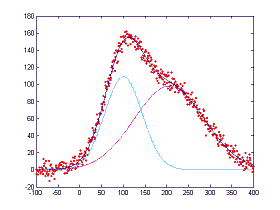fitting function fitgauss.m. In this case there are two non-linear parameters: peak position and peak width (the peak height is a linear parameter and is determined by regression in a single step in line 9 of the fitting function fitgauss.m and is returned in the global variable "c"). Compared to the simpler polynomial least-squares methods for measuring peaks, the iterative method has the advantage of using all the data points across the entire peak, including zero and negative points, and it can be applied to multiple overlapping peaks as demonstrated in in Demofitgauss2.m (shown on the left).

To accommodate the possibility that the baseline may shift, we can add a column of 1s to the A matrix, just as was done in the CLS method. This has the effect of introducing an additional "peak" into the model that has an amplitude but no position or width. The baseline amplitude is returned along with the peak heights in the global vector “c”; Demofitgaussb.m and fitgauss2b.m illustrates this addition. (Demofitlorentzianb.m and fitlorentzianb.m for Lorentzian peaks).

This peak fitting technique is easily extended to any number of overlapping peaks of the same type using the same fitting function fitgauss.m, which easily adapts to any number of peaks, depending on the length of the first-guess "start" vector lambda that is passed to the function as input arguments, along with the data vectors t and y:

```1 function err = fitgauss(lambda,t,y)
2 %   Fitting functions for a Gaussian band spectrum.
3 %  T. C. O'Haver, 2006   Updated to Matlab 6, March 2006
4 global c
5 A = zeros(length(t),round(length(lambda)/2));
6 for j = 1:length(lambda)/2,
7     A(:,j) = gaussian(t,lambda(2*j-1),lambda(2*j))';
8 end
9 c = A\y'; %  c = abs(A\y') for positive peak heights only
10 z = A*c;
11 err = norm(z-y');```
If there are n peaks in the model, then the length of lambda is 2n, one entry for each iterated variable ([position1 width1 position2 width2....etc]). The "for" loop (lines 5-7) constructs a n × length(t) matrix containing the model for each peak separately, using a user-defined peak shape function (in this case gaussian.m), then it computes the n-length peak height vector c by least-squares regression in line 9, using the Matlab shortcut "\" notation. (To constrain the fit to positive values of peak height, replace A\y' with abs(A\y') in line 9). The resulting peak heights are used to compute z, the sum of all n model peaks, by matrix multiplication in line 10, and then "err", the root-mean-square difference between the model z and the actual data y, is computed in line 11 by the Matlab 'norm' function and returned to the calling function ('fminsearch'), which repeats the process many times, trying different values of the peak positions and the peak widths until the value of "err" is low enough.

This fitting function above would be called by Matlab's fminsearch function like so
:

params=fminsearch(@(lambda)(fitgauss(lambda,x,y)),[50 20])
,

where the square brackets contain a vector of first guesses for position and width for each peak
([position1 width1 position2 width2....etc]). The output argument 'params' returns a 2 × n matrix of  best-fit values of position and width for each peak, and the peak heights are contained in the n-length global variable vector c. Similar fitting functions can be defined for other peak shapes simply by calling the corresponding peak shape function, such as lorentzian.m in line 7.  (Note: in order for this and other scripts like Demofitgauss.m or Demofitgauss2.m to work on your version of Matlab, all the functions that they call must be loaded into Matlab beforehand, in this case fitgauss.m and gaussian.m. Scripts that call sub-functions must have those functions in the Matlab path. Functions, on the other hand, can have all their required sub-functions defined within the main function itself and thus can be self-contained, as are the next two examples).

fitshape2.m
([Positions,Heights,Widths,FittingError]=fitshape2(x,y,start)) pulls all of this together into
a simplified general-purpose Matlab/Octave function for fitting multiple overlapping model shapes to the data contained in the vector variables x and y. The model is the weighted sum of any number of basic peak shapes which are defined mathematically as a function of x, with two variables that the program will independently determine for each peak, positions and widths, in addition to the peak heights (i.e. the weights of the weighted sum). You must provide the first-guess starting vector 'start', in the form  [position1 width1 position2 width2 ...etc], which specifies the first-guess position and width of each component (one pair of position and width for each peak in the model). The function returns the parameters of the best-fit model in the vectors Positions,Heights,Widths, and computes the percent error between the data and the model in FittingError. It also plots the data as dots and the fitted model as a line.
The interesting thing about this function is that the only part that defines the shape of the model is the last line. In fitshape2.m, that line contains the expression for a Gaussian peak of unit height, but you could change that to any other expression or algorithm that computes g as a function of x with two unknown parameters 'pos' and 'wid' (position and width, respectively, for peak-type shapes, but they could represent anything for other function types, such as the exponential pulse, sigmoidal, etc.); everything else in the fitshape.m function can remain the same.
It's all about the bottom line. This makes fitshape a good platform for experimenting with different mathematical expression as proposed models to fit data. There are also two other variations of this function for models with one iterated variable plus peak height (fitshape1.m) and three iterated variables plus peak height (fitshape3.m). Each has illustrative examples in the help file (type "help fitshape...").Variable shape types, such as the Voigt profile, Pearson, Breit-Wigner-Fano, Gauss-Lorentz blend, and the exponentially-broadened Gaussian and Lorentzian, are defined not only by a peak position, height, and width, but also by an additional parameter that fine-tunes the shape of the peak. If that parameter is equal and known for all peaks in a group, it can be passed as an additional input argument to the shape function, as shown in VoigtFixedAlpha.m for the Voigt profile (which is calculated as a convolution of Gaussian and Lorentzian components with different widths). If the shape parameter is allowed to be different for each peak in the group and is to be determined by iteration (just as is position and width), then the routine must be modified to accommodate three, rather than two, iterated variables, as shown in VoigtVariableAlpha.m. Although the fitting error is lower with variable alphas, the execution time is longer and the alphas values so determined are not very stable, with respect to noise in the data and the starting guess values, especially for multiple peaks. (These are self-contained functions). Version 9.5 of the general-purpose Matlab/Octave function peakfit.m includes fixed and variable shape types for the Pearson, ExpGaussian, Voigt, and Gaussian/Lorentzian blend, as well as the 3-parameter logistic or Gompertz function (whose three parameters are labeled Bo, Kh, and L, rather than position, width, and shape factor). The script VoigtShapeFittingDemonstration.m uses the peakfit.m version 9.5 to fit a single Voigt profile and to calculate the Gaussian width component, Lorentzian width component, and alpha. It computes the theoretical Voigt profile and adds random noise for realism. VoigtShapeFittingDemonstration2.m does the same for two overlapping Voigt profiles, using both fixed alpha and variable alpha models (shape numbers 20 and 30). (Requires voigt.m, halfwidth.m, and peakfit.m in the path).

If you do not know the shape of your peaks, you can use use peakfit.m or ipf.m to try different shapes to see if one of the standard shapes included in those programs fits the data; try to find a peak in your data that is typical, isolated, and that has a good signal-to-noise ratio. For example, the Matlab functions ShapeTestS.m and ShapeTestA.m tests the data in its input arguments x,y, assumed to be a single isolated peak, fits it with different candidate model peak shapes using peakfit.m, plots each fit in a separate figure window, and prints out a table of fitting errors in the command window. ShapeTestS.m tries seven different candidate symmetrical model peaks, and ShapeTestA.m tries six different candidate asymmetrical model peaks. The one with the lowest fitting error (and R2 closest to 1.000) is presumably the best candidate. Try the examples in the help files for each of these functions. But beware, if there is too much noise in your data, the results can be misleading. For example, even if the actual peak shape is something other than a Gaussian, the multiple Gaussians model is likely to fit slightly better because it has more degrees of freedom and can "fit the noise". The Matlab function peakfit.m has many more built-in shapes to choose from, but still it is a finite list and there is always the possibility that the actual underlying peak shape is not available in the software you are using or that it is simply not describable by a mathematical function.

Signals with peaks of different shape types in one signal can be fit by the fitting function fitmultiple.m, which takes as input arguments a vector of peak types and a vector of shape variables. The sequence of peak types and shape parameters must be determined beforehand. To see how this is used, see Demofitmultiple.m.

You can create your own fitting functions for any purpose; they are not limited to single algebraic expressions, but can be arbitrarily complex multiple step algorithms. For example, in the TFit method for quantitative absorption spectroscopy, a model of the instrumentally-broadened transmission spectrum is fit to the observed transmission data, using a fitting function that performs Fourier convolution of the transmission spectrum model with the known slit function of the spectrometer. The result is an alternative method of calculating absorbance that allows the optimization of signal-to-noise ratio and extends the dynamic range and calibration linearity of absorption spectroscopy far beyond the normal limits.

The effect of random noise on the uncertainty of the peak parameters determined by iterative least-squares fitting is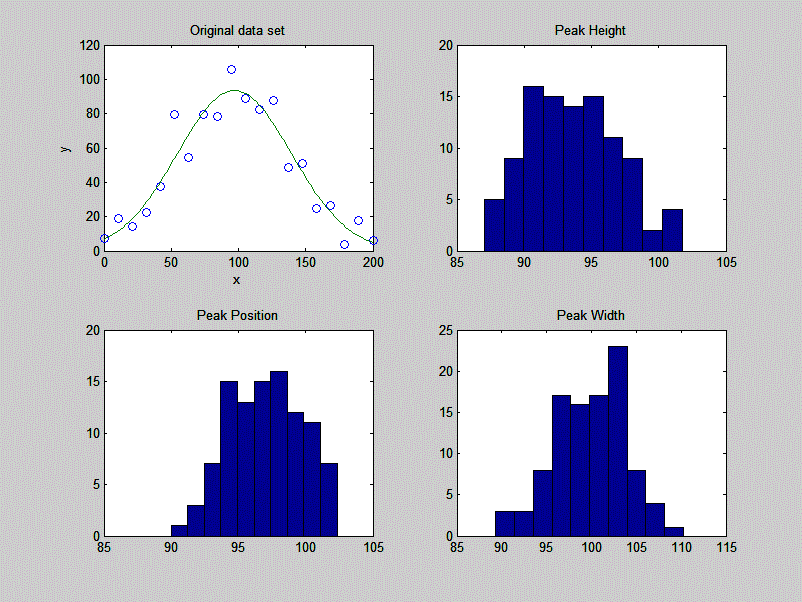readily estimated by the bootstrap sampling method, covered in a previous section, which randomly assigns weights of 0, 1, and 2 to the data points. A simple demonstration of bootstrap estimation of the variability of an iterative least-squares fit to a single noisy Gaussian peak is given by the custom downloadable Matlab/Octave function "BootstrapIterativeFit.m", which creates a single x,y data set consisting of a single noisy Gaussian peak, extracts bootstrap samples from that data set, performs an iterative fit to the peak on each of the bootstrap samples, and plots the distributions (histograms) of peak height, position, and width of the bootstrap samples. The syntax is BootstrapIterativeFit(TrueHeight, TruePosition, TrueWidth, NumPoints, Noise, NumTrials) where TrueHeight is the true peak height of the Gaussian peak, TruePosition is the true x-axis value at the peak maximum, TrueWidth is the true half-width (FWHM) of the peak, NumPoints is the number of points taken for the least-squares fit, Noise is the standard deviation of (normally-distributed) random noise, and NumTrials is the number of bootstrap samples.  An typical example for BootstrapIterativeFit(100,100,100,20,10,100); is displayed in the figure on the right.

>> BootstrapIterativeFit(100,100,100,20,10,100);
Peak Height  Peak Position  Peak Width
mean:   99.27028    100.4002       94.5059
STD:    2.8292      1.3264         2.9939
IQR:    4.0897      1.6822         4.0164
IQR/STD Ratio:    1.3518

A similar demonstration function for two overlapping Gaussian peaks is available in "BootstrapIterativeFit2.m".  Type "help BootstrapIterativeFit2" for more information.  In both these simulations, the standard deviation (STD) as well as the interquartile range (IQR) of each of the peak parameters are computed. This is done because the interquartile range is much less influenced by outliers. The distribution of peak parameters measured by iterative fitting is often non-normal, exhibiting a greater fraction of large deviations from the mean than is expected for a normal distribution. This is because the iterative procedure sometimes converges on an abnormal result, especially for multiple peak fits with a large number of variable parameters. (You may be able to see this in the histograms plotted by these simulations, especially for the weaker peak in BootstrapIterativeFit2).  In those cases the standard deviation will be too high because of the outliers, and the IQR/STD ratio will be much less than the value of 1.34896 that is expected for a normal distribution. In that case a better estimate of the standard deviation of the central portion of the distribution (without the outliers) is IQR/1.34896.

It's important to emphasize that the bootstrap method predicts only the effect of random noise on the peak parameters for a fixed fitting model. It does not take into account the possibility of peak parameter inaccuracy cased by using a non-optimum data range, or choosing an imperfect model, or by inaccurate compensation for the background/baseline, all of which are at least partly subjective and thus beyond the range of influences that can easily be treated by random statistics. If the data have relatively little random noise, or have been smoothed to reduce the noise, then it's likely that model selection and baseline correction will be the major sources of peak parameter inaccuracy, which are not well predicted by the bootstrap method.

For the quantitative measurement of peaks, it's instructive to compare the iterative least-squares method with simpler, less computationally-intensive, methods. For example, the measurement of the peak height of a single peak of uncertain width and position could be done simply by taking the maximum of the signal in that region. If the signal is noisy, a more accurate peak height will be obtained if the signal is smoothed beforehand. But smoothing can distort the signal and reduce peak heights. Using an iterative peak fitting method, assuming only that the peak shape is known, can give the best possible accuracy and precision, without requiring smoothing even under high noise conditions, e.g. when the signal-to-noise ratio is 1, as in the demo script SmoothVsFit.m:

True peak height = 1    NumTrials = 100    SmoothWidth = 50
Method        Maximum y    Max Smoothed y    Peakfit
Average peak height   3.65         0.96625         1.0165
Standard deviation    0.36395      0.10364         0.11571

If peak area is measured rather than peak height, smoothing is unnecessary (unless to locate the peak beginning and end) but peak fitting still yields the best precision. See SmoothVsFitArea.m.

It's also instructive to compare the iterative least-squares method with classical least-squares curve fitting, discussed in the previous section, which can also fit peaks in a signal.  The difference is that in the classical least squares method, the positions, widths, and shapes of all the individual components are all known beforehand; the only unknowns are the amplitudes (e.g. peak heights) of the components in the mixture.  In non-linear iterative curve fitting, on the other hand, the positions, widths, and heights of the peaks are all unknown beforehand; the only thing that is known is the fundamental underlying shape of the peaks.  The non-linear iterative curve fitting is more difficult to do (for the computer, anyway) and more prone to error, but it's necessary if you need to track shifts in peak position or width or to decompose a complex overlapping peak signal into fundamental components knowing only their shape. The Matlab/Octave script “CLSvsINLS.m” compares the classical least-squares (CLS) method with three different variations of the iterative method (INLS) method for measuring the peak heights of three Gaussian peaks in a noisy test signal, demonstrating that the fewer the number of unknown parameters, the faster and more accurate is the peak height calculation.

Method  Positions  Widths       Execution time  Accuracy
CLS     known     known         0.00133        0.30831
INLS    unknown   unknown       0.61289        0.6693
INLS    known     unknown       0.16385        0.67824
INLS    unknown   known         0.24631        0.33026
INLS    unknown   known (equal) 0.15883        0.31131

Another comparison of multiple measurement techniques is presented in Case Study D.

### Peak Fitter for Matlab and Octave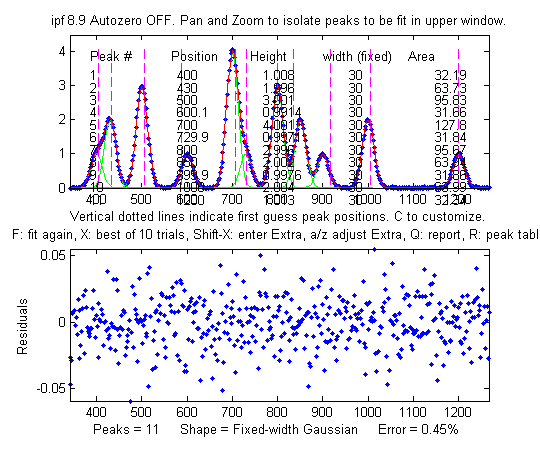Peak fitting functions and scripts that uses an unconstrained non-linear optimization algorithm to decompose a complex, overlapping-peak time-series signal into its component peaks. The objective is to determine whether your signal can be represented as the sum of fundamental underlying peaks shapes. These functions accept signals of any length, including those with non-integer and non-uniform x-values, and can fit any number of peaks with Gaussian, equal-width Gaussian, fixed-width Gaussian, exponentially-broadened Gaussian, exponentially-broadened equal-width Gaussians, bifurcated Gaussian, Lorentzian, fixed-width Lorentzians, equal-width Lorentzians, exponentially-broadened Lorentzian, bifurcated Lorentzian, logistic distribution, logistic function, triangular, alpha function, Pearson 7, exponential pulse, up sigmoid, down sigmoid, Gaussian/Lorentzian blend, Breit-Wigner-Fano, and Voigt profile shapes. Here is a graphic that illustrates the basic peak shapes available.

There are two different versions, a command line version called peakfit.m, for Matlab or Octave, and a keypress operated interactive version called ipf.m, for Matlab only. For adding as an element on your own programs and for automating the fitting of large numbers of signals, peakfit.m is better; ipf.m is best for exploring a few signals to determine the best fitting range, peak shapes, number of peaks, baseline correction mode, etc. Both functions allow for simplified operation by providing default values for any unspecified input arguments; for example, the starting values, if not specified in the input arguments, are estimated by the program based on the length and x-axis interval of the data. Compared to the fitshape.m function described above, peakfit.m has a large number of built-in peak shapes, it does not require (although it can be given) the first-guess position and width of each component, and it has features for background correction and other useful features to improve the quality and reliability of fits.

The latest versions of these functions can optionally estimate the expected standard deviation and interquartile range of the peak parameters using the bootstrap sampling method.  See DemoPeakfitBootstrap for a self-contained demo of this function.

### Accuracy and precision of peak parameter measurement (with examples in Matlab)[Model errors]  [Number of peaks]  [Peak width]  [Background correction]  [Random noise]  [Iterative fitting errors]  [Exponential broadening]  [Effect of smoothing]

Iterative curve fitting is often used to measure the position, height, and width of peaks in a signal, especially when they overlap significantly. There are four major sources of error in measuring these peak parameters by iterative curve fitting: model errors, background correction, random noise, and iterative fitting errors.  This section makes use of the downloadable peakfit.m function. Instructions are here or type "help peakfit". (Once you have peakfit.m in youjr path, you can simply copy and paste, or drag and drop, any of the following single-line or multi-line code examples into the Matlab or Octave editor or into the command line and press Enter to execute it).

a. Model errorsPeak shape.
If you have the wrong model for your peaks, the results can not be expected to be accurate; for instance, if your actual peaks are Lorentzian in shape, but you fit them with a Gaussian model, or vice versa. For example, a single isolated Gaussian peak at x=5, with a height of 1.000 fits a Gaussian model virtually perfectly, using the Matlab user-defined peakfit function, as shown on the right. (The 5th input argument for the peakfit function specifies the shape of peaks to be used in the fit; "1" means Gaussian).

>> x=[0:.1:10];y=exp(-(x-5).^2);
>> [FitResults,MeanFitError]=peakfit([x' y'],5,10,1,1)

Peak#     Position     Height      Width        Area
FitResults =

1            5            1       1.6651       1.7725
MeanFitError =         R2=
7.8579e-07            1

The "FitResults" are, from left to right, peak number, peak position, peak height, peak width, and peak area. The MeanFitError, or just "fitting error", is the square root of  the sum of the squares of the differences between t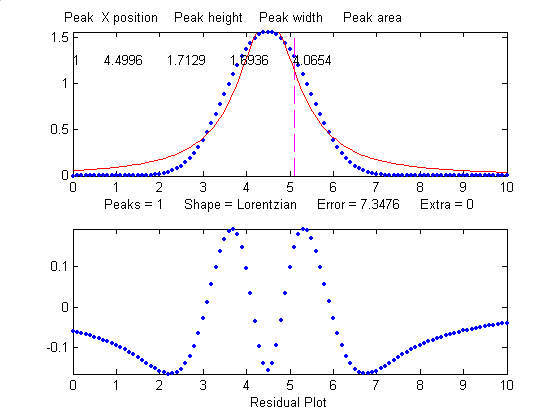he data and the best-fit model, as a percentage of the maximum signal in the fitted region.  R2 is the "R-squared" or coefficient of determination, which is exactly 1 for a perfect fit. Note the good agreement of the area measurement (1.7725) with the theoretical area under the curve of exp(-x2), which turns out to be the square root of pi, or about 1.7725....

But this same peak, when fit with the incorrect model (a Logistic model, peak shape number 3), gives a fitting error of 1.4% and height and width errors of 3% and 6%, respectively. However, the peak area error is only 1.7%, because the height and width errors partially cancel out. So you don't have to have a perfect model to get a decent area measurement.

>> [FitResults,MeanFitError]=peakfit([x' y'],5,10,1,3)
Peak#     Position     Height      Width          Area
FitResults =
1       5.0002      0.96652        1.762       1.7419
MeanFitError =1.4095

When fit with an even more incorrect Lorentzian model (peak shape 2, shown on the right), this peak gives a 6% fitting error and height, width and area errors of 8%, 20%, and 17%, respectively.

>> [FitResults,MeanFitError]=peakfit([x' y'],5,10,1,2)
FitResults =
Peak#     Position    Height      Width         Area

1            5       1.0876       1.3139       2.0579
MeanFitError =5.7893

But, practically speaking, Gaussian and Lorentzian shapes are so visually distinct that it's unlikely that your estimate of a model will be that far off. Real peak shapes are often some unknown combination of peak shapes, such as Gaussian with a little Lorentzian or vice versa, or some slightly asymmetrical modification of a standard symmetrical shape. So if you use an available model that is at least close to the actual shape, the parameters errors may not be so bad and may in fact be better than other measurement methods.

So clearly the larger the fitting errors, the larger are the parameter errors, but the parameter errors are of course not equal to the fitting error (that would just be too easy).  Also, it's clear that the peak height and width are the parameters most susceptible to errors. The peak positions, as you can see here, are measured accurately even if the model is way wrong, as long as the peak is symmetrical and not highly overlapping with other peaks.

A good fit is not by itself proof that the shape function you have chosen is the correct one; in some cases the wrong function can give a fit that looks perfect. For example, this fit of a real data set to a 5-peak Gaussian model has a low percent fitting error and the residuals look random - usually an indicator of a good fit. But in fact in this case the model is wrong; that data came from an experimental domain where the underlying shape is fundamentally non-Gaussian but in some cases can look very like a Gaussian. As another example, a data set consisting of peaks with a Voigt profile peak shape can be fit with a  weighted sum of a Gaussian and a Lorentzian almost as well as an with an actual Voigt model, even though those models are not the same mathematically; the difference in fitting error is so small that it would likely be obscured by the random noise if it were a real experimental signal. The same thing can occur in sigmoidal signal shapes: a pair of simple 2-parameter logistic functions seems to fit this example data pretty well, with a fitting error of less than 1%; you would have no reason to doubt the goodness of fit unless the random noise is low enough so you can see that the residuals are wavy. Alternatively, a 3-parameter logistic (Gompertz function) fits much better, and the residuals are random, not wavy. In such cases you can not depend solely on what looks like a good fit to determine whether the fit is model is optimum; sometimes you need to know more about the peak shape expected in that kind of experiment, especially if the data are noisy. At best, if you do get a good fit with random non-wavy residuals, you can claim that the data are consistent with the proposed model. Note: with the peakfit.m function, you can extract the residuals as a vector by using the syntax [FitResults,GOF,baseline,coeff,residual,xi,yi]=peakfit(....

Sometimes the accuracy of the model is not so important. In quantitative analysis applications, where the peak height or areas measured by curve fitting is used only to determine the concentration of the substance that created the peak by constructing a calibration curve, using laboratory prepared standards solutions of known concentrations, the necessity of using the exact peak model is lessened, as long as the shape of the unknown peak is constant and independent of concentration. If the wrong model shape is used, the R2 for curve fitting will be poor (much less than 1.000) and the peak heights and areas measured by curve fitting will be inaccurate, but the error will be exactly the same for the unknown samples and the known calibration standards, so the error will cancel out and, as a result, the R2 for the calibration curve can be very high (0.9999 or better) and the measured concentrations will be no less accurate than they would have been with a perfect peak shape model. Even so, it's useful to use as accurate a model peak shape as possible, because the R2 for curve fitting will work better as a warning indicator if something unexpected goes wrong during the analysis (such as an increase in the noise or the appearance of an interfering peak from a foreign substance). See PeakShapeAnalyticalCurve.m for a Matlab/Octave demonstration.

Number of peaks. Another source of model error occurs if you have the wrong number of peaks in your model, for example if the signal actually hastwo peaks but you try to fit it with only one peak. In the example below, a line of Matlab code generates a simulated signal with of two Gaussian peaks at x=4 and x=6 with peaks heights of 1.000 and 0.5000 respectively and widths of 1.665, plus random noise with a standard deviation 5% of the height of the largest peak (a signal-to-noise ratio of 20):

>>x=[0:.1:10];y=exp(-(x-6).^2)+.5*exp(-(x-4).^2)+.05*randn(size(x));

In a real experiment you would not usually know the peak positions, heights, and widths; you would be using curve fitting to measure those parameters. Let's assume that, on the basis of previous experience or some preliminary trial fits, you have established that the optimum peak shape model is Gaussian, but you don't know for sure how many peaks are in this group. If you start out by fitting this signal with a single-peak Gaussian model, you get:

>> [FitResults,MeanFitError]=peakfit([x' y'],5,10,1,1)

FitResults
Peak#   Position   Height     Width      Area
1       5.5291     0.86396    2.9789    2.7392
MeanFitError = 10.467The residual plot shows a "wavy" structure that's visible in the random scatter of points due to the random noise in the signal. This means that the fitting error is not limited by the random noise; it is a clue that the model is not quite right.

But a fit with two peaks yields much better results (The 4th input argument for the peakfit function specifies the number of peaks to be used in the fit).

>> [FitResults,MeanFitError]=peakfit([x' y'],5,10,2,1)
FitResults =
Peak#    Position  Height    Width    Area

1      4.0165     0.50484   1.6982   0.91267
2      5.9932     1.0018    1.6652   1.7759
MeanFitError =4.4635

Now the residuals have a random scatter of points, as would be expected if the signal is accurately fit except for the random noise. Moreover, the fitting error is much lower (less that half) of the error with only one peak. In fact, the fitting error is just about what we would expect in this case based on the 5% random noise in the signal (estimating the relative standard deviation of the points in the baseline visible at the edges of the signal). Because this is a simulation in which we know beforehand the true values of the peak parameters (peaks at x=4 and x=6 with peaks heights of 1.0 and 0.50 respectively and widths of 1.665), we can actually calculate the parameter errors (the difference between the real peak positions, heights, and widths and the measured values). Note that they are quite accurate (in this case within about 1% relative on the peak height and 2% on the widths), which is actually better than the 5% random noise in this signal because of the averaging effect of fitting to multiple data points in the signal.

But if going from one peak to two peaks gave us a better fit, why not go to three peaks?  If there were no noise in the data, and if the underlying peak shape were perfectly matched by the model, then the fitting error would have already been essentially zero with two model peaks, and adding a third peak to the model would yield a vanishingly small height for that third peak. But in our examples here, as in real data, there is always some random noise, and the result is that the third peak height will not be zero. Changing the number of peaks to three gives these results:

>> [FitResults,MeanFitError]=peakfit([x' y'],5,10,3,1)
FitResults =
Peak#  Position   Height    Width     Area

1    4.0748     0.51617   1.7874    0.98212
2    6.7799     0.089595  2.0455    0.19507
3    5.9711     0.94455   1.53      1.5384
MeanFitError =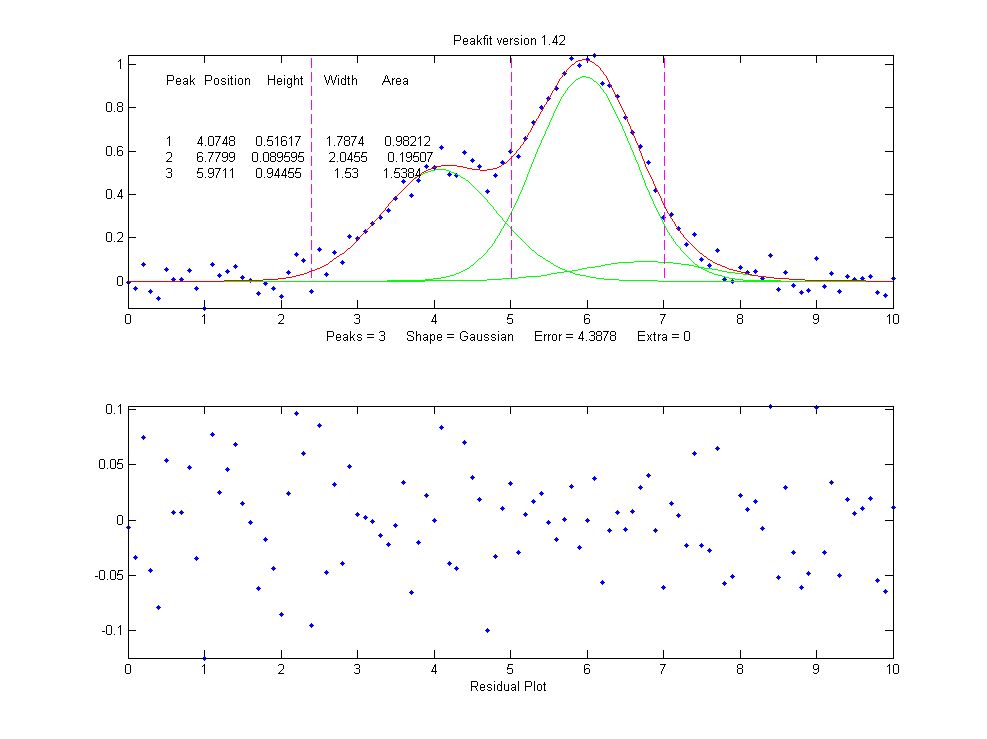4.3878

The fitting algorithm has now tried to fit an additional low-amplitude peak (numbered peak 2 in this case) located at x=6.78. The fitting error is actually lower that for the 2-peak fit, but only slightly lower, and the residuals are no less visually random that with a 2-peak fit.  So, knowing nothing else, a 3-peak fit might be rejected on that basis alone. In fact, there is a serious downside to fitting more peaks than are actually present in the signal: it increases the parameter measurement errors of the peaks that are actually present. Again, we can prove this because we know beforehand the true values of the peak parameters: clearly the peak positions, heights, and widths of the two real peaks than are actually in the signal (peaks 1 and 3) are significantly less accurate than those of the 2-peak fit.

Moreover, if we repeat that fit with the same signal but with a different sample of random noise (simulating a repeat measurement of a stable experimental signal in the presence or random noise), the additional third peak in the 3-peak fit will bounce around all over the place (because the third peak is actually fitting the random noise, not an actual peak in the signal).

>> x=[0:.1:10];
>> y=exp(-(x-6).^2)+.5*exp(-(x-4).^2)+.05*randn(size(x));
>> [FitResults,MeanFitError]=peakfit([x' y'],5,10,3,1)
FitResults =

Peak#   Position    Height     Width      Area

1       4.115      0.44767     1.8768     0.89442
2       5.3118     0.09340     2.6986     0.26832
3       6.0681     0.91085     1.5116     1.4657
MeanFitError = 4.4089

With this new set of data, two of the peaks (numbers 1 and 3) have roughly the same position, height, and width, but peak number 2 has changed substantially compared to the previous run. Now we have an even more compelling reason to reject the 3-peak model: the 3-peak solution is not stable. And because this is a simulation in which we know the right answers, we can verify that the accuracy of the peak heights is substantially poorer (about 10% error) than expected with this level of random noise in the signal (5%). If we were to run a 2-peak fit on the same new data, we get much better measurements of the peak heights.

>> [FitResults,MeanFitError]=peakfit([x' y'],5,10,2,1)
FitResults =
Peak#   Position    Height     Width     Area
1      4.1601      0.49981    1.9108    1.0167
2      6.0585      0.97557    1.548     1.6076
MeanFitError = 4.4113

If this is repeated several times, it turns out that the peak parameters of the peaks at x=4 and x=6 are, on average, more accurately measured by the 2-peak fit. In practice, the best way to evaluate a proposed fitting model is to fit several repeat measurements of the same signal (if that is practical experimentally) and to compute the standard deviation of the peak parameter values.

In real experimental work, of course, you usually don't know the right answers beforehand, so that's why it's important to use methods that work well when you do know. The real data example mentioned above was fit with a succession of 2, 3, 4 and 5 Gaussian models, until the residuals became random. Beyond that point, there is little to be gained by adding more peaks to the model. Another way to determine the minimum number of models peaks needed is to plot the fitting error vs the number of model peaks; the point at which the fitting error reaches a minimum, and increases afterward, would be the fit with the "ideal combination of having the best fit without excess/unnecessary terms". The Matlab/Octave function testnumpeaks.m (R = testnumpeaks(x, y, peakshape, extra, NumTrials, MaxPeaks)) applies this idea by fitting the x,y data to a series of models of shape peakshape containing 1 to MaxPeaks model peaks. The correct number of underlying peaks is either the model with the lowest fitting error, or, if two or more models have about the same fitting error, the model with the least number of peaks. The Matlab/Octave demo script NumPeaksTest.m uses this function with noisy computer-generated signals containing a user-selected 3, 4, 5 or 6 underlying peaks. With very noisy data, however, the technique is not always reliable.

Peak width constraints. Finally, there is one more thing that we can do that might improve the peak parameter measurement accuracy, and it concernsthe peak widths. In all the above simulations, the basic assumption that all the peak parameters were unknown and independent of one another. In some types of measurements, however, the peak widths of each group of adjacent peaks are all expected to be equal to each other, on the basis of first principles or previous experiments. This is a common situation in analytical chemistry, especially in atomic spectroscopy and in chromatography, where the peak widths are determined largely by instrumental factors.

In the current simulation, the true peaks widths are in fact both equal to 1.665, but all the results above show that the measured peak widths are close but not quite equal, due to random noise in the signal. The unequal peak widths are a consequence of the random noise, not real differences in peak width. But we can introduce an equal-width constraint into the fit by using peak shape 6 (Equal-width Gaussians) or peak shape 7 (Equal-width Lorentzians). Using peak shape 6 on the same set of data as the previous example:

>> [FitResults,MeanFitError]=peakfit([x' y'],5,10,2,6)
FitResults =

Peak#  Position   Height    Width    Area
1     4.0293     0.52818   1.5666   0.8808
2     5.9965     1.0192    1.5666   1.6997
MeanFitError =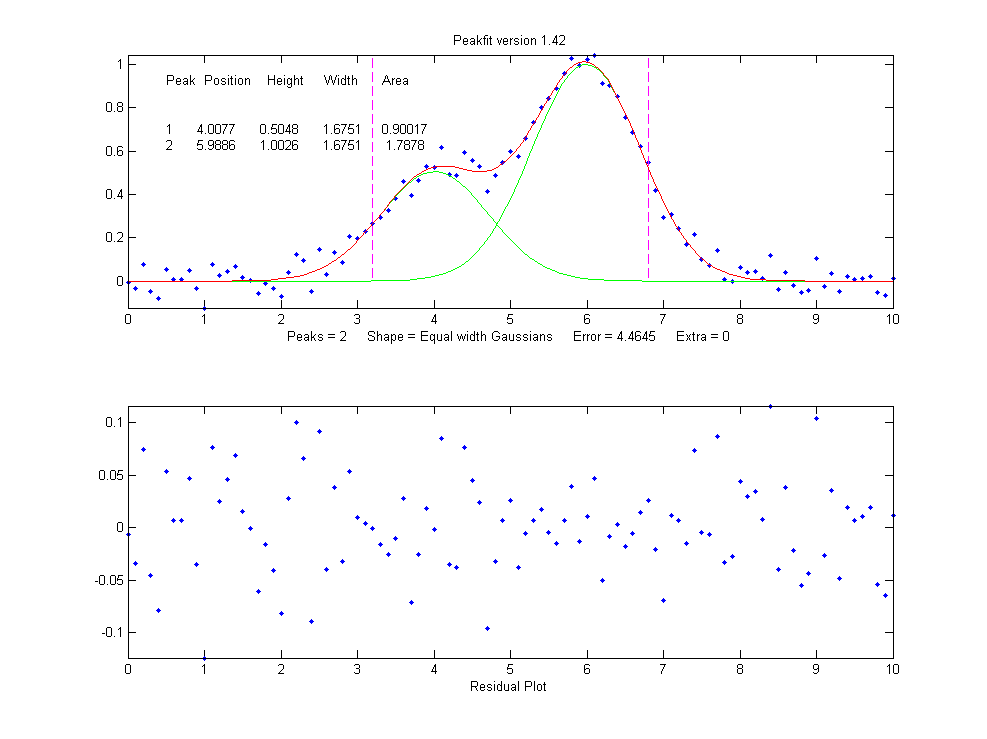4.5588

This "equal width" fit forces all the peaks within one group to have exactly the same width, but that width is determined by the program from the data. The result is a slightly higher fitting error (in this case 4.5% rather than 4.4%), but - perhaps surprisingly - the peak parameter measurements are usually more accurate and more reproducible (Specifically, the relative standard deviations are on average lower for the equal-width fit than for an unconstrained-width fit to the same data, assuming of course that the true underlying peak widths are really equal). This is an exception to the general expectation that lower fitting errors result in lower peak parameter errors. It is an illustration of the general rule that the more you know about the nature of your signals, and the closer your chosen model adheres to that knowledge, the better the results. In this case we knew that the peak shape was Gaussian (although we could have verified that choice by trying other candidate peaks shapes). We determined that the number of peaks was 2 by inspecting the residuals and fitting errors for 1, 2, and 3 peak models. And then we introduced the constraint of equal peak widths within each group of peaks, based on prior knowledge of the experiment rather than on inspection of residuals and fitting errors. Here's another example, with real experimental data from a measurement where the peak widths are expected to be equal, showing the result of an unconstrained fit and an equal width fit; the fitting errors is slightly larger for the equal-width fit, but that is to be preferred in this case. Not every experiment can be expected to yield peaks of equal width, but when it does, it's better to make use of that constraint.

Fixed-width shapes. Going one step beyond equal widths (in peakfit version 7.6 and later), you can also specify a fixed-width shapes (shape numbers 11, 12, 34-37), in which the width of the peaks are known beforehand, but are not necessarily equal, and are specified as a vector in input argument 10, one element for each peak, rather than being determined from the data as in the equal-width fit above. Introducing this constraint onto the previous example, and supplying an accurate width as the 10th input argument:

>> [FitResults,MeanFitError]=peakfit([x' y'],0,0,2,11,0,0,0,0,[1.666 1.666])
FitResults =
Peak#     Position     Height      Width       Area

1       3.9943      0.49537      1.666      0.8785
2       5.9924      0.98612      1.666      1.7488
MeanFitError = 4.8128

Comparing to the previous equal-width fit, the fitting error of 4.8% is larger here (because there are fewer degrees of freedom to minimize the error), but the parameter errors, particularly the peaks heights, are more accurate because the width information provided in the input argument was more accurate (1.666) than the width determined by the equal-width fit (1.5666). Again, not every experiment yields peaks of known width, but when it does, it's better to make use of that constraint. For example, see Example 35 and the Matlab/Octave script WidthTest.m (typical results for a Gaussian/Lorentzian blend shape shown below, showing that the more constraints, the greater the fitting error but the lower the parameter errors, if the constraints are accurate).

 Relative percent error Fitting error Position Error Height Error Width Error Unconstrained shape factor and widths: shape 33 0.78 0.39 0.80 1.66 Fixed shape factor and variable widths: shape 13 0.79 0.25 1.3 0.98 Fixed shape factor and fixed widths: shape 35 0.8 0.19 0.69 0.0

Multiple linear regression (peakfit version 9). Finally, note that if the peak positions are also known, and only the peak heights are unknown, you don't even need to use the iterative fitting method at all; you can use the easier and faster multilinear regression technique (also called “classical least squares”) which is implemented by the function cls.m and by version 9 of peakfit.m as shape number 50. Although multilinear regression results in fitting error slightly greater (and R2 lower), the errors in the measured peak heights are often less, as in this example from peakfit9demo.m, where the true peak heights of the three overlapping Gaussian peaks are 10, 30, and 20.
Multilinear regression results (known position and width):
Peak    Position     Height       Width   Area
1         400      9.9073         70     738.22
2         500      29.995         85     2714
3         560      19.932         90     1909.5
%fitting error=1.3048   R2= 0.99832   %MeanHeightError=0.427

Unconstrained iterative non-linear least squares results:
Peak   Position     Height      Width    Area
1      399.7      9.7737      70.234    730.7
2      503.12     32.262      88.217    3029.6
3      565.08     17.381      86.58     1601.9
%fitting error=1.3008   R2= 0.99833   %MeanHeightError=7.63

This demonstrates dramatically how different measurement methods can look the same, and give fitting errors almost the same, and yet differ greatly in parameter measurement accuracy. (The similar script peakfit9demoL.m is the same thing with Lorentzian peaks).

SmallPeak.m is a demonstration script comparing all these techniques applied to the challenging problem of measuring the height of a small peak that is closely overlapped with and completely obscured by a much larger peak. It compares unconstrained, equal-width, and fixed-position iterative fits (using peakfit.m) with a classical least squares fit in which only the peak heights are unknown (using cls.m). It helps to spread out the four figure windows so you can observe the dramatic difference in stability of the different methods. A final table of relative percent peak height errors shows that the more the constraints, the better the results (but only if the constraints are justified). The real key is to know which parameters can be relied upon to be constant and which have to be allowed to vary.

Here's a a screen video (MorePeaksLowerFittingError.mp4) of a real-data experiment using the interactive peak fitter ipf.m with a complex experimental signal in which several different fits were performed using models from 4 to 9 variable-width, equal-width, and fixed-width Gaussian peaks. The fitting error gradually decreases from 11% initially to 1.4% as more peaks are used, but is that really justified? If the objective is simply to get a good fit, then do whatever it takes. But if the objective is to extract some useful information from the model peak parameters, then more specific knowledge about that particular experiment is needed: how many peaks are really expected; are the peak widths really expected to be constrained? Note that in this particular case the residuals (bottom panel) are never really random and always have a distinct "wavy" character, suggesting that the data may have been smoothed before curve fitting (usually not a good idea: see http://wmbriggs.com/blog/?p=195). Thus there is a real possibility that some of those 9 peaks are simply "fitting the noise", as will be discussed further in Appendix A.

b. Background correction.The peaks that are measured in many scientific instruments are sometimes superimposed on a non-specific background or baseline. Ordinarily the experiment protocol is designed to minimize the background or to compensate for the background, for example by subtracting a "blank" signal from the signal of an actual specimen. But even so there is often a residual background that can not be eliminated completely experimentally. The origin and shape of that background depends on the specific measurement method, but often this background is a broad, tilted, or curved shape, and the peaks of interest are comparatively narrow features superimposed on that background. In some cases the baseline may be another peak. The presence of the background has relatively little effect on the peak positions, but it is impossible to measure the peak heights, width, and areas accurately unless something is done to account for the background.

There are various methods described in the literature for estimating and subtracting the background in such cases. The simplest assumption is that the background can be approximated as a simple function in the local region of group of peaks being fit together, for example as a constant (flat), straight line (linear) or curved line (quadratic). This is the basis of the "autozero" modes in the ipf.m, iSignal.m, and iPeak.m functions, which are selected by the T key to cycle thorough OFF, linear, quadratic, and flat modes. In the flat mode, a constant baseline is included in the curve fitting calculation, as described above. In linear mode, a straight-line baseline connecting the two ends of the signal segment in the upper panel will be automatically subtracted before the iterative curve fitting. In quadratic mode, a parabolic baseline is subtracted. In the last two modes, you must adjust the pan and zoom controls to isolate the group of overlapping peaks to be fit, so that the signal returns to the local background at the left and right ends of the window.

Example of an experimental chromatographic signal. From left to right, (1) Raw data with peaks superimposed on a tilted baseline. One group of peaks is selected using the the pan and zoom controls, adjusted so that the signal returns to the local background at the edges of the segment displayed in the upper window; (2) The linear baseline is subtracted when the autozero mode set to 1 in ipf.m by pressing the T key; (3) Fit with a three-peak Gaussian model, activated by pressing 3, G, F (3 peaks, Gaussian, Fit).

Alternatively, it may be better to subtract the background from the entire signal first, before further operations are performed. As before, the simplest assumption is that the background is piece-wise linear, that is, can be approximated as a series of small straight line segments.  This is the basis of the multiple point background subtraction mode in ipf.m, iPeak.m, and in iSignal.  The user enters the number of points that is thought to be sufficient to define the baseline, then clicks where the baseline is thought to be along the entire length of the signal in the lower whole-signal display (e.g. on the valleys between the peaks).  After the last point is clicked, the program interpolates between the clicked points and subtracts the piece-wise linear background from the original signal.

From left to right, (1) Raw data with peaks superimposed on baseline. (2) Background subtracted from the entire signal using the multipoint background subtraction function in iPeak.m (ipf.m and iSignal have the same function).

Sometimes, even without an actual baseline present, the peaks may overlap enough so that the signal never return to the baseline, making it seem that there is a baseline to be corrected. This can occur especially with peaks shapes that have gradually sloping sides, such as the Lorentzian, as shown in this example. Curve fitting without baseline correction will work in that case.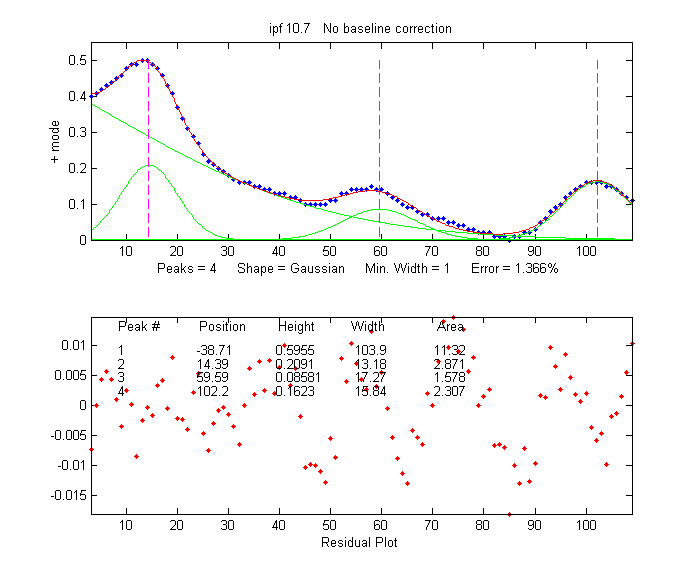In many cases the background may be modeled as a broad peak whose maximum falls outside of the range of data acquired, as in the real-data example on the left. It may be possible to fit the off-screen peak simply by including an extra peak in the model to account for the baseline. In the example on the left, there are three clear peaks visible, superimposed on a tilted baseline. In  this case the signal was fit nicely with four, rather than three, variable-width Gaussians, with an error of only 1.3%. The additional broad Gaussian, with a peak at  x = -38.7, serves as the baseline. (Obviously, you shouldn't use the equal-width shapes for this, because the background peak is broader than the other peaks).

In another real-data example of an experimental spectrum, the linear baseline subtraction ("autozero") mode described above is used in conjunction with a 5-Gaussian model, with one Gaussian component fitting the broad peak that may be part of the background and the other four fitting the sharper peaks. This fits the data very well (0.5% fitting error), but a fit like this can be difficult to get, because there are so many other solutions with slightly higher fitting errors; it may take several trials. It can help if you specify the start values for the iterated variables, rather than using the default choices; all the software programs described here have that capability.

The Matlab/Octave function peakfit.m can employ a peakshape input argument that is a vector of different shapes, which can be useful for baseline correction. As an example, consider a weak Gaussian peak on sloped straight-line baseline, using a 2-component fit with one Gaussian component and one variable-slope straight line ('slope', shape 26), specified by using the vector [1 26] as the shape argument:

x=8:.05:12;y=x+exp(-(x-10).^2);
[FitResults,GOF]= peakfit([x;y],0,0,2,[1 26],[1 1],1,0)
FitResults =
1         10          1     1.6651     1.7642
2      4.485    0.22297       0.05     40.045
GOF =
0.0928    0.9999
If the baseline seems to be curved rather than straight, you can model the baseline with a quadratic (shape 46) rather than a linear slope (peakfit version 8 and later).

If the baseline seems to be different on either side of the peak, you can try to model the baseline with an S-shape (sigmoid), either an up-sigmoid, shape 10 (click for graphic), peakfit([x;y],0,0,2,[1 10],[0 0], or a down-sigmoid, shape 23 (click for graphic), peakfit([x;y],0,0,2,[1 23],[0 0], in these examples leaving the peak modeled as a Gaussian.

If the signal is very weak compared to the baseline, the fit can be helped by adding rough first guesses ('start') using the 'polyfit' function to generate automatic first guesses for the sloping baseline. For example, with two overlapping signal peaks and a 3-peak fit with peakshape=[1 1 26].

x=4:.05:16;
y=x+exp(-(x-9).^2)+exp(-(x-11).^2)+.02.*randn(size(x));
start=[8 1 10 1 polyfit(x,y,1)];
peakfit([x;y],0,0,3,[1 1 26],[1 1 1],1,start)

A similar technique can be employed in a spreadsheet, as demonstrated in

The downside to including the baseline as a variable component is that it increases the number of degrees of freedom, increases the execution time, and increases the possibility of unstable fits. Specifying start values can help.

c. Random noise in the signal.Any experimental signal has a certain amount of random noise, which means that the individual data points scatter randomly above and below their mean values.  The assumption is ordinarily made that the scatter is equally above and below the true signal, so that the long-term average approaches the true mean value; the noise "averages to zero" as it is often said. The practical problem is that any given recording of the signal contains only one finite sample of the noise.  If another recording of the signal is made, it will contain another independent sample of the noise. These noise samples are not infinitely long and therefore do not represent the true long-term nature of the noise. This presents two problems: (1) an individual sample of the noise will not "average to zero" and thus the  parameters of the best-fit model will not necessarily equal the true values, and (2) the magnitude of the noise during one sample might not be typical; the noise might have been randomly greater or smaller than average during that time. This means that the mathematical "propagation of error" methods, which seek to estimate the likely error in the model parameters based on the noise in the signal, will be subject to error (underestimating the error if the noise happens to be lower than average and overestimating the errors if the noise happens to be larger than average).

A better way to estimate the parameter errors is to record multiple samples of the signal, fit each of those separately, compute the models parameters from each fit, and calculate the standard error of each parameter. But if that is not practical, it is possible to simulate such measurements by adding random noise to a model with known parameters, then fitting that simulated noisy signal to determine the parameters, then repeating the procedure over and over again with different sets of random noise. This is exactly what the script DemoPeakfit.m (which requires the peakfit.m function) does for simulated noisy peak signals such as those illustrated below. It's easy to demonstrate that, as expected, the average fitting error precision and the relative standard deviation of the parameters increases directly with the random noise level in the signal. But the precision and the accuracy of the measured parameters also depend on which parameter it is (peak positions are always measured more accurately than their heights, widths, and areas) and on the peak height and extent of peak overlap (the two left-most peaks in this example are not only weaker but also more overlapped than the right-most peak, and therefore exhibit poorer parameter measurements). In this example, the fitting error is 1.6% and the percent relative standard deviation of the parameters ranges from 0.05% for the peak position of the largest peak to 12% for the peak area of the smallest peak.

Overlap matters: The errors in the values of peak parameters measured by curve fitting depend not only on the characteristics of the peaks in question and the signal-to-noise ratio, but also upon other peaks that are overlapping it.  From left to right: (1) a single peak at x=100 with a peak height of 1.0 and width of 30 is fit with a Gaussian model, yielding a relative fit error of 4.9% and relative standard deviation of peak position, height, and width of  0.2%, 0.95%, and 1.5% , respectively. (2) The same peak, with the same noise level but with another peak overlapping it, reduces the relative fit error to 2.4% (because the addition of the second peak increases overall signal amplitude), but increases the relative standard deviation of peak position, height, and width to 0.84%, 5%, and 4% - a seemingly better fit, but with poorer precision for the first peak. (3) The addition of a third (non-overlapping) peak reduces the fit error to 1.6% , but the relative standard deviation of peak position, height, and width of the first peak are still 0.8%, 5.8%, and 3.64%, about the same as with two peaks, because the third peak does not overlap the first one significantly.If the average noise noise in the signal is not known or its probability distribution is uncertain, it is possible to use the bootstrap sampling method to estimate the uncertainty of the peak heights, positions, and widths, as illustated on the left and as described in detail above. The latest version of the keypress operated interactive version of ipf.m has added a function (activated by the 'v' key) that estimates the expected standard deviation of the peak parameters using this method.

One way to reduce the effect of noise is to take more data. If the experiment makes it possible to reduce the x-axis interval between points, or to take multiple readings at each x-axis values, then the resulting increase in the number of data points in each peak should help reduce the effect of noise. As a demonstration, using the script DemoPeakfit.m to create a simulated overlapping peak signal like that shown above right, it's possible to change the interval between x values and thus the total number of data points in the signal. With a noise level of 1% and 75 points in the signal, the fitting error is 0.35 and the average parameter error is 0.8%. With 300 points in the signal and the same noise level, the fitting error is essentially the same, but the average parameter error drops to 0.4%, suggesting that the accuracy of the measured parameters varies inversely with the square root of the number of data points in the peaks.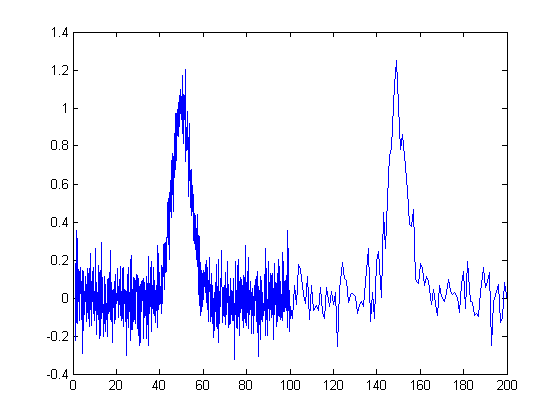The figure on the right illustrates the importance of sampling interval and data density. You can download the data file "udx" in TXT format or in Matlab MAT format. The signal consists of two Gaussian peaks, one located at x=50 and the second at x=150. Both peaks have a peak height of 1.0 and a peak half-width of 10, and normally-distributed random white noise with a standard deviation of 0.1 has been added to the entire signal. The x-axis sampling interval, however, is different for the two peaks; it's 0.1 for the first peak and 1.0 for the second peak. This means that the first peak is characterized by ten times more points than the second peak. When you fit these peaks separately to a Gaussian model (e.g., using peakfit.m or ipf.m), you will find that all the parameters of the first peak are measured more accurately than the second, even though the fitting error is not much different:

First peak:                                                      Second peak:
Percent Fitting Error=7.6434%  Percent Fitting Error=8.8827%
Peak# Position Height Width    Peak# Position  Height  Width
1      49.95   1.0049 10.111   1     149.64   1.0313   9.941

So far this discussion has applied to white noise. But other noise colors have different effects. Low-frequency weighted (“pink”) noise has a greater effect on the accuracy of peak parameters measured by curve fitting, and, in a nice symmetry, high-frequency “blue” noise has a smaller effect on the accuracy of peak parameters that would be expected on the basis of its standard deviation, because the information in a smooth peak signal is concentrated at low frequencies. An example of this occurs when curve fitting is applied to a signal that has been deconvoluted to remove a broadening effect. This is why smoothing before curve fitting does not help, because the peak signal information is concentrated in the low frequency range, but smoothing reduces mainly the noise in the high frequency range.

Sometime you may notice that the residuals in a curve fitting operation are structured into bands or lines rather than being completely random. This can occur if either the independent variable or the dependent variable is quantized into discrete steps rather than continuous. It may look strange, but it has little effect on the results as long as the random noise is larger than the steps.

When there is noise in the data (in other words, pretty much always), the exact results will depend on the region selected for the fit - for example, the results will vary slightly with the pan and zoom setting in ipf.m, and the more noise, the greater the effect.

d. Iterative fitting errors.Unlike multiple linear regression curve fitting, iterative methods may not always converge on the exact same model parameters each time the fit is repeated with slightly different starting values (first guesses). The Interactive Peak Fitter ipf.m makes it easy to test this, because it uses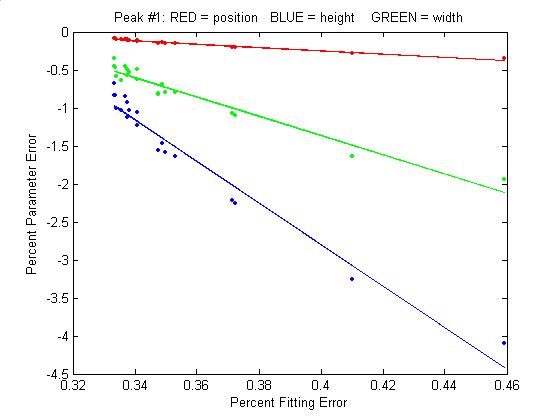slightly different starting values each time the signal is fit (by pressing the F key in ipf.m, for example). Even better, by pressing the X key, the ipf.m function silently computes 10 fits with different starting values and takes the one with the lowest fitting error. A basic assumption of any curve fitting operation is that the fitting error (the root-mean-square difference between the model and the data) is minimized, the parameter errors (the difference between the actual parameters and the  parameters of the best-fit model) will also be minimized. This is generally a good assumption, as demonstrated by the graph to the right, which shows typical percent parameters errors as a function of fitting error for the left-most peak in one sample of the simulated signal generated by DemoPeakfit.m (shown in the previous section). The variability of the fitting error here is caused by random small variations in the first guesses, rather than by random noise in the signal.  In many practical cases there is enough random noise in the signals that the iterative fitting errors within one sample of the signal are small compared to the random noise errors between samples.

Remember that the variability in measured peak parameters from fit to fit of a single sample of the signal is not a good estimate of the precision or accuracy of those parameters, for the simple reason that those results represent only one sample of the signal, noise, and background. The sample-to-sample variations are likely to be much greater than the within-sample variations due to the iterative curve fitting. (In this case, a "sample" is a single recording of signal). To estimate the contribution of random noise to the variability in measured peak parameters when only a single sample if the signal is available, the bootstrap method can be used.

e. Selecting the optimum data region of interest.  When you perform a peak fitting using ipf.m, you have control over data region selected by using the pan and zoom controls (or, using the command-line function peakfit.m, by setting the center and window input arguments). Changing these settings usually changes the resulting fitted peak parameters. If the data were absolutely perfect, say, a mathematically perfect peak shape with no random noise, then the pan and zoom settings would make no difference at all; you would get the exact same values for peak parameters at all settings, assuming only that the model you are using matches the actual shape. But of course in the real world, data are never mathematically perfect and noiseless. The greater the amount of random noise in the data, or the greater the discrepancy between your data and the model you select, the more the measured parameters will vary if you fit different regions using the pan and zoom controls. This is simply an indication of the uncertainty in the measured parameters.

f. A difficult caseAs a dramatic example of the ideas in parts c and d, consider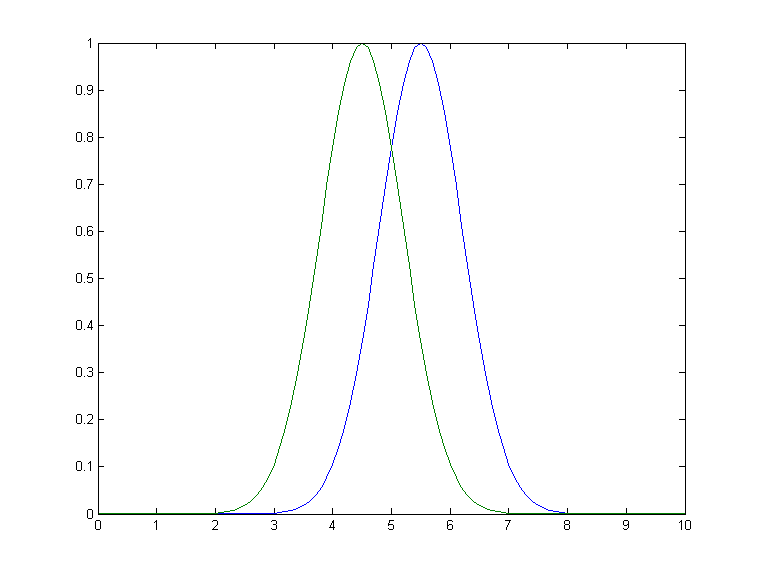this simulated example signal, consisting of two Gaussian peaks of equal height = 1.00, overlapping closely enough so that their sum is a single symmetrical peak that looks very much like a single Gaussian.

>> x=[0:.1:10]';
>> y=exp(-(x-5.5).^2)+exp(-(x-4.5).^2);

If there were no noise in the signal, the peakfit.m or ipf.m routines could easily extract the two equal Gaussian components to an accuracy of 1 part in 1000.

>> peakfit([x y],5,19,2,1)

Peak#   Position   Height     Width    Area
1      4.5004    1.001     1.6648    1.773

2      5.5006    0.99934   1.6641    1.770

But in the presence of even a little noise (for example, 1% RSD), the results are uneven; one peak is almost always significantly higher than the other:

>> y=exp(-(x-5.5).^2)+exp(-(x-4.5).^2)+.01*randn(size(x))
>> peakfit([x y],5,19,2,1)

Peak#   Position    Height     Width    Area
1      4.4117     0.83282    1.61     1.43
2      5.4022     1.1486     1.734    2.12

The fit is stable with any one sample of noise (if peakfit.m was run again with slightly different starting values, for example by pressing the F key several times in ipf.m), so the problem is not iterative fitting errors caused by different starting values. The problem is the noise: although the signal is completely symmetrical, any particular sample of the noise is not perfectly symmetrical (e.g. the first half of the noise usually averages a slightly higher or lower than the second half, resulting in an asymmetrical fit result). The surprising thing is that the error in the peak heights are much larger (about 15% relative, on average) than the random noise in the data (1% in this example). So even though the fit looks good - the fitting error is low (less than 1%) and the residuals are random and unstructured - the model parameters can still be very far off. If you were to make another measurement (i.e. generate another independent set of noise), the results would be different but still inaccurate (the first peak has an equal chance of being larger or smaller than the second). Unfortunately, the expected error is not accurately predicted by the bootstrap method, which seriously underestimates the standard deviation of the peak parameters with repeated measurements of independent signals (because a bootstrap sub-sample of asymmetrical noise is likely to remain asymmetrical). A Monte Carlo simulation would give a more reliable estimation of uncertainty in such cases.

Better results can be obtained in cases where the peak widths are expected to be equal, in which case you can use peak shape 6 (equal-width Gaussian) instead of peak shape 1: peakfit([x y],5,19,2,6). It also helps to provide decent first guesses (start) and to set the number of trials (NumTrials) to a number above 1): peakfit([x,y],5,10,2,6,0,10,[4 2 5 2],0,0). The best case will be if the shape, position, and width of the two peaks are known accurately, and if the only unknown is their heights. Then the Classical Least Squares (multiple regression) technique can be employed and the results will be much better.

Appendix AE illustrates one way to deal with the problem of excessive peak overlap in a multi-step script that uses first-derivative symmetrization as a pre-process performed before iterative least-squares curve fitting to analyze a complex signal consisting of multiple asymmetric overlapping peaks. This results in better peak parameter accuracy, even though the fitting error is no better.

For an even more challenging example like this, where the two closely overlapping peak are very different in height, see Appendix Q.

So, to sum up, we can make the following observations about the accuracy of model parameters: (1) the parameter errors depend on the accuracy of the model chosen and on number of peaks; (2) the parameter errors are directly proportional to the noise in the data (and worse for low-frequency or pink noise); (3) all else being equal, parameter errors are proportional to the fitting error, but a model that fits the underlying reality better, e.g. equal or fixed widths or shapes) often gives lower parameter errors even if the fitting error is larger; (4) the errors are typically least for peak position and worse for peak width and area; (5) the errors depend on the data density (number of independent data points in the width of each peak) and on the extent of peak overlap (the parameters of isolated peaks are easier to measure than highly overlapped peaks); (6) if only a single signal is available, the effect of noise on the standard deviation of the peak parameters in many cases can be predicted approximately by the bootstrap method, but if the overlap of the peaks is too great, the error of the parameter measurements can be much greater than predicted.

Fitting signals that are subject to exponential broadening.

DataMatrix2 (figure on the right) is a computer-generated test signal consisting of 16 symmetrical Gaussian peaks with random whi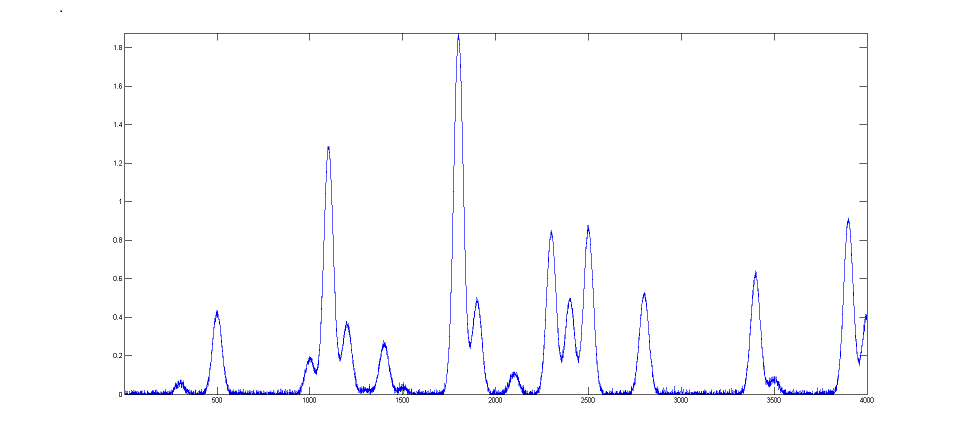te noise added. The peaks occur in groups of 1, 2, or 3 overlapping peaks, but the peak maxima are located at exactly integer values of x from 300 to 3900 (on the 100's) and the peak widths are always exactly 60 units. The peak heights vary from 0.06 to 1.85. The standard deviation of the noise is 0.01. You can use this signal to test curve-fitting programs and to determine the accuracy of their measurements of peak parameters. Right-click and select "Save" to download this signal, put it in the Matlab path, then type "load DataMatrix2" at the command prompt to load it into the Matlab workspace.

DataMatrix3 (figure on the left) i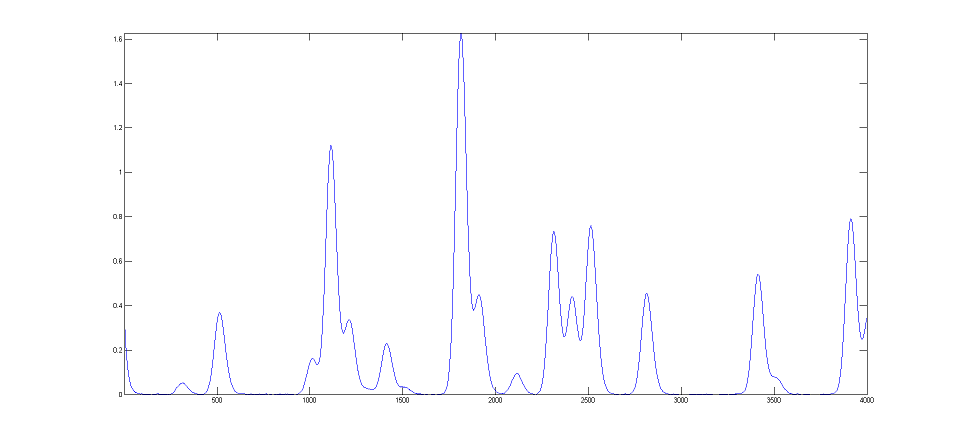s a exponentially broadened version of DataMatrix2, with a "decay constant", also called "time constant", of 33 points on the x-axis.  The result of the exponential broadening is that all the peaks in this signal are asymmetrical, their peak maxima are shifted to longer x values, and their peak heights are smaller and their peak widths are larger than the corresponding peaks in DataMatrix2. Also, the random noise is damped in this signal compared to the original and is no longer "white", as a consequence of the broadening. This type of effect is common in physical measurements and often arises from some physical or electrical effect in the measurement system that is apart from the fundamental peak characteristics. In such cases it is usually desirable to compensate for the effect of the broadening, either by deconvolution or by curve fitting, in an attempt to measure what the peak parameters would have been before the broadening (and also to measure the broadening itself).  This can be done for Gaussian peaks that are exponentially broadened by using the "ExpGaussian" peak shape in peakfit.m and ipf.m (or the "ExpLorentzian", if the underlying peaks are Lorentzian).  Right-click and select "Save" to download this signal, put it in the Matlab path, then type "load DataMatrix3" to load it into the Matlab workspace.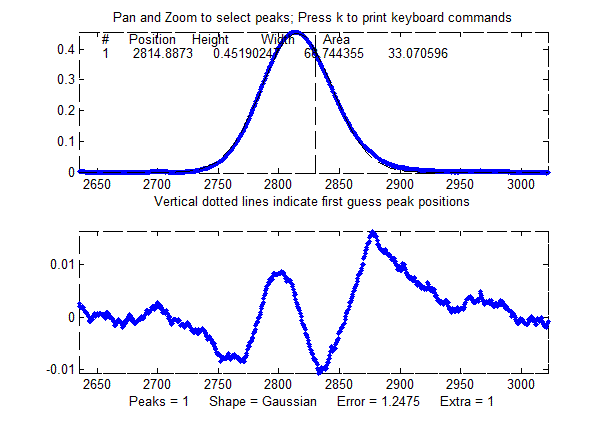The example illustrated on the right focuses on the single isolated peak whose "true" peak position, height, width, and area in the original unbroadened signal, are 2800, 0.52, 60, and 33.2 respectively. (The relative standard deviation of the noise is 0.01/0.52=2%.) In the broadened signal, the peak is visibly asymmetrical, the peak maximum is shifted to larger x values, and it has a shorter height and larger width, as demonstrated by the attempt to fit a normal (symmetrical) Gaussian to the broadened peak. (The peak area, on the other hand, is  not much effected by the broadening).

DataMatrix3

>> ipf(DataMatrix3);

Peak Shape = Gaussian
Autozero ON
Number of peaks = 1
Fitted range = 2640 - 2979.5 (339.5)  (2809.75)
Percent Error = 1.2084
Peak# Position  Height      Width      Area
1     2814.832  0.45100549  68.441262  32.859436

The large "wavy" residual in the plot above is a tip-off that the model is not quite right. Moreover, the fitting error (1.2%) is larger than expected for a peak with a half-width of 60 points and a 2% noise RSD (approximately 2%/sqrt(60)=0.25%).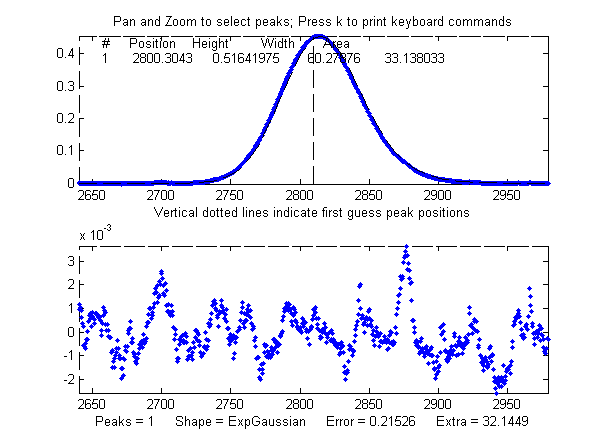Fitting to an exponentially-broadened Gaussian (pictured on the right) gives a much lower fitting error ("Percent error") and a more nearly random residual plot. But the interesting thing is that it also recovers the original peak position, height, and width to an accuracy of a fraction of 1%. In performing this fit, the decay constant ("extra") was experimentally determined from the broadened signal by adjusting it with the A and Z keys to give the lowest fitting error; that also results in a reasonably good measurement of the broadening factor (32.6, vs the actual value of 33). Of course, had the original signal been nosier, these measurements would not be so accurate. Note: When using peakshape 5 (fixed decay constant exponentially broadened Gaussian) you have to give it a reasonably good value for the  decay constant ('extra'), the input argument right after the peakshape number.  If the value is too far off, the fit may fail completely, returning all zeros. A little trial and error suffice. (Also, peakfit.m version 8.4 has two forms of unconstrained variable decay constant exponentially-broadened Gaussian, shape numbers 31 and 39, that will measure the decay constant as an iterated variable. Shape 31 creates the shape by performing a Fourier convolution of a specified Gaussian by an exponential decay of specified decay constant, whereas shape 39 (expgaussian2.m) uses a mathematical expression for the final shape so produced. Both result in the same peak shape but are parameterized differently. Shape 31 reports the peak height and position as that of the original Gaussian before broadening, whereas shape 39 reports the peak height of the broadened result. Shape 31 reports the width as the FWHM (full width at half maximum) and shape 39 reports the standard deviation (sigma) of the Gaussian. Shape 31 reports the exponential factor an the number of data points and shape 39 reports the reciprocal of decay constant in time units. (See the script DemoExpgaussian.m for a more detailed numerical example). For multiple-peak fits, both shapes usually require a reasonable first guess ('start") vector for best results. If the exponential decay constant of each peak is expected to be different and you need to measure those values, use shapes 31 or 39, but the decay constant of all the peaks is expected to be the same, use shape 5, and determine the decay constant by fitting an isolated peak. For example:

Autozero ON
Number of peaks = 1
Extra = 32.6327
Fitted range = 2640 - 2979.5 (339.5)  (2809.75)
Percent Error = 0.21696
Peak#  Position   Height      Width      Area
1      2800.1302  0.51829906  60.086295  33.152429

Comparing the two methods, the
exponentially-broadened Gaussian fit recovers all the underlying peak parameters quite accurately:

 Position Height Width Area Actual peak parameters 2800 0.52 60 33.2155 Gaussian fit to broadened signal 2814.832 0.45100549 68.441262 32.859436 ExpGaussian fit to broadened signal 2800.1302 0.51829906 60.086295 33.152429

Other peaks in the same signal, under the broadening influence of the same decay constant, can be fit with similar settings, for example the set of three overlapping peaks near x=2400.  As before, the peak positions are recovered almost exactly and even the width measurements are reasonably accurate (1% or better). If the exponential broadening decay constant is not the same for all the peaks in the signal, for example if it gradually increases for larger x values, then the decay constant setting can be optimized for each group of peaks.

The smaller fitting error evident here is just a reflection of the larger peak heights in this particular group of peaks - the noise is the same everywhere in this signal.

Autozero OFF
Number of peaks = 3
Extra = 31.9071
Fitted range = 2206 - 2646.5 (440.5)  (2426.25)
Percent Error = 0.11659
Peak# Position    Height      Width       Area
1     2300.2349  0.83255884   60.283214   53.422354
2     2400.1618  0.4882451    60.122977   31.24918
3     2500.3123  0.85404245   60.633532   55.124839

The residual plots in both of these examples still have some "wavy" character, rather than being completely random and "white".  The exponential broadening smooths out any white noise in the original signal that is introduced before the exponential effect, acting as a low-pass filter in the time domain and resulting in a low-frequency dominated "pink" noise, which is what remains in the residuals after the broadened peaks have been fit as well as possible. On the other hand, white noise that is introduced after the exponential effect would continue to appear white and random on the residuals.  In real experimental data, both types of noise may be present in varying amounts.One final caveat: peak asymmetry similar to exponential broadening could possibly be the result a pair of closely-spaced peaks of different peak heights.  In fact, a single exponential broadened Gaussian peak can sometimes be fit with two symmetrical Gaussians to a fitting error at least as low as a single exponential broadened Gaussian fit. This makes it hard to distinguish between these two models on the basis of fitting error alone. However, this can usually be decided by inspecting the other peaks in the signal: in most experiments, exponential broadening applies to every peak in the signal, and the broadening is either constant or changes gradually over the length of the signal. On the other hand, it is relatively unlikely that every peak in the signal will be accompanied by a smaller side peak that varies in this way. So, if a only one or a few of the peaks exhibit asymmetry, and the others are symmetrical, it's most likely that the asymmetry is due to closely-spaced peaks of different peak heights. If all peaks have the same or similar asymmetry, it's more likely to be a broadening factor that applies to the entire signal. The two figures here provide an example from real experimental data. On the left, three asymmetrical peaks are each fit with two symmetrical Gaussians (six peaks total). On the right, those three peaks are fit with one exponentially broadened Gaussian each (three peaks total). In this case, the three asymmetrical peaks all have the same asymmetry and can be fit with the same decay constant ("extra"). Moreover, the fitting error is slightly lower for the three-peak exponentially broadened fit. Both of these observations argue for the three-peak exponentially broadened fit rather than the six-peak fit.

Note: if your peaks are trailing off to the left, rather that to the right as in the above examples, simply use a negative value for the decay constant (in ipf.n, press Shift-X and type a negative values).

An alternative to this type of curve fitting for exponential broadened peaks is to use the first-derivative addition technique to remove the asymmetry and then fit the resulting peak with a symmetrical model. This is faster in terms of computer execution time, especially for signals with many peaks, but it requires that the exponential time constant be known or estimated experimentally beforehand.

The Effect of Smoothing before least-squares analysis.In general, it is not advisable to smooth a signal before applying least-squares fitting, because doing so might distort the signal, can make it hard to evaluate the residuals properly, and might bias the results of bootstrap sampling estimations of precision, causing it to underestimate the between-signal variations in peak parameters. SmoothOptimization.m is a Matlab/Octave script that compares the effect of smoothing on the measurements of peak height of a Gaussian peak with a half-width of 166 points, plus white noise with a signal-to-noise ratio (SNR) of 10, using three different methods:
(a) simply taking the single point at the center of the peak as the peak height;
(b) using the gaussfit method to fit the top half of the peak (see CurveFitting.html#Transforming), and
(c) fitting the entire signal with a Gaussian using the iterative method.
The results of 150 trials with independent white noise samples are shown on the left: a typical raw signal is shown in the upper left. The other three plots show the effect of the SNR of the measured peak height vs the smooth ratio (the ratio of the smooth width to the half-width of the peak) for those three measurement methods. The results show that the simple single-point measurement is indeed much improved by smoothing, as is expected; however, the optimum SNR (which improves by roughly the square root of the peak width of 166 points) is achieved only when the smooth ratio approaches 1.0, and that much smoothing distorts the peak shape significantly, reducing the peak height by about 40%. The curve-fitting methods are much less effected by smoothing and the iterative method hardly at all. So the bottom line is that you should not smooth prior to curve-fitting, because it will distort the peak and will not gain any significant SNR advantage. The only situations where it might be advantageous so smooth before fitting are when the noise in the signal is high-frequency weighted (i.e. "blue" noise), where low-pass filtering will make the peaks easier to see for the purpose of setting the staring points for an iterative fit, or if the signal is contaminated with high-amplitude narrow spike artifacts, in which case a median-based pre-filter can remove the spikes without much change to the rest of the signal. And, in another application altogether, if you want to fit a curve joining the successive peaks of a modulated wave (called the "envelope"), then you can smooth the absolute value of the wave before fitting the envelope.This page is part of "A Pragmatic Introduction to Signal Processing", created and maintained by Prof. Tom O'Haver , Department of Chemistry and Biochemistry, The University of Maryland at College Park. Comments, suggestions and questions should be directed to Prof. O'Haver at toh@umd.edu.  Last updated September, 2020.